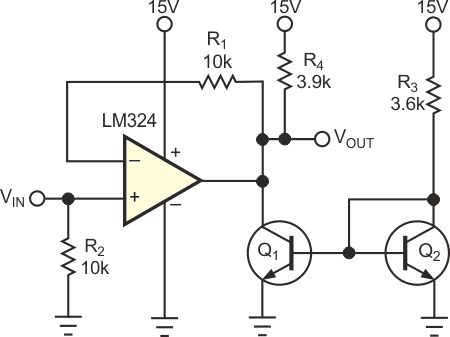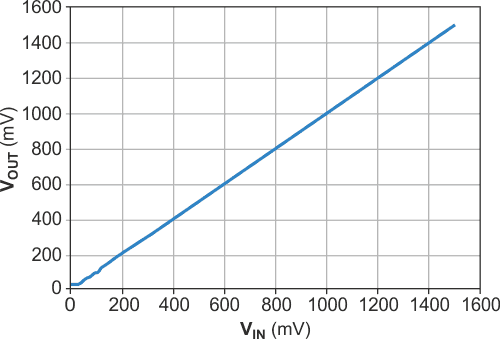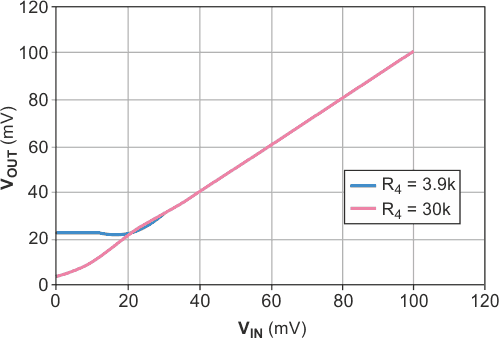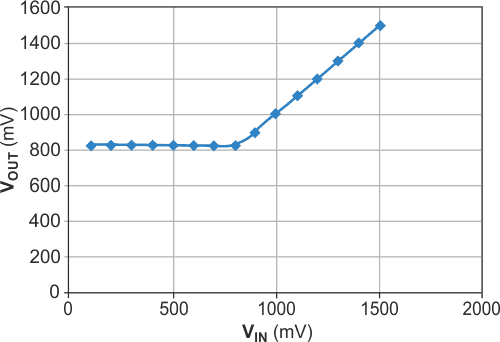# Current source enables op amp's output to go to ground

## Texas Instruments LM324

The LM324 is a cost-effective choice for an op amp, especially when you need to apply ground-level inputs. Although its output purportedly includes ground, its poor current-sinking capability limits the applications. At output voltages lower than 0.5 V, the op amp's sinking current ranges only from 2 to 100 µA. You can add an external current-sinking circuit to bring the usable output voltage down to the millivolt level.Figure 1. An external current source can bring the usable output level of an LM324 down to the millivolt level.Figure 2. The transfer function of Figure 1’s circuit is linear down to the low-millivolt level.

In Figure 1, Q1, Q2, and R3 form a 4-mA current source that drains the output of the LM324. R4 is the load, demanding a sink current of 4 mA. This design uses a 2N2222 transistor for its low saturation voltage. The output characteristic becomes the saturation characteristic of the added transistors, Q1 and Q2. Using this current source, the output voltage is linear down to 22 mV above ground. Figure 2 and Figure 3 show the output characteristics. The lowest usable output voltage depends on the load (sink) current. When the load current is 0.5 mA (R4 = 30 kΩ), the output voltage is linear down to 4 mV.Figure 3. With 0.5-mA load current, the output voltage is linear down to 4 mV.Figure 4. This graphic shows the LM324 transfer function without the added current source.

Figure 4 is the original output characteristic of the LM324 driving R4 (3.9 kΩ) without the added sinking current source. The current source presents a constant load to the LM324. You can configure a leftover op amp as a voltage comparator to cut off the current source when the output voltage is higher than 1 V.

## Materials on the topic

EDNYou may have to register before you can post comments and get full access to forum.
 User Name Remember Me? Password